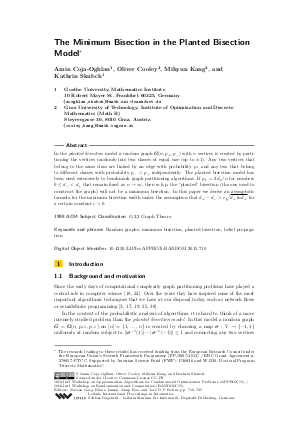Document# The Minimum Bisection in the Planted Bisection Model

### Authors Amin Coja-Oghlan, Oliver Cooley, Mihyun Kang, Kathrin Skubch## File

LIPIcs.APPROX-RANDOM.2015.710.pdf
• Filesize: 0.49 MB
• 16 pages

## Cite As

Amin Coja-Oghlan, Oliver Cooley, Mihyun Kang, and Kathrin Skubch. The Minimum Bisection in the Planted Bisection Model. In Approximation, Randomization, and Combinatorial Optimization. Algorithms and Techniques (APPROX/RANDOM 2015). Leibniz International Proceedings in Informatics (LIPIcs), Volume 40, pp. 710-725, Schloss Dagstuhl - Leibniz-Zentrum für Informatik (2015)
https://doi.org/10.4230/LIPIcs.APPROX-RANDOM.2015.710

## Abstract

In the planted bisection model a random graph G(n,p_+,p_-) with n vertices is created by partitioning the vertices randomly into two classes of equal size (up to plus or minus 1). Any two vertices that belong to the same class are linked by an edge with probability p_+ and any two that belong to different classes with probability (p_-) <(p_+) independently. The planted bisection model has been used extensively to benchmark graph partitioning algorithms. If (p_+)=2(d_+)/n and (p_-)=2(d_-)/n for numbers 0 <= (d_-) <(d_+) that remain fixed as n tends to infinity, then with high probability the "planted" bisection (the one used to construct the graph) will not be a minimum bisection. In this paper we derive an asymptotic formula for the minimum bisection width under the assumption that (d_+)-(d_-) > c * sqrt((d_+)ln(d_+)) for a certain constant c>0.
##### Keywords
• Random graphs
• minimum bisection
• planted bisection
• belief propagation.

## Metrics

• Access Statistics
• Total Accesses (updated on a weekly basis)
0